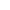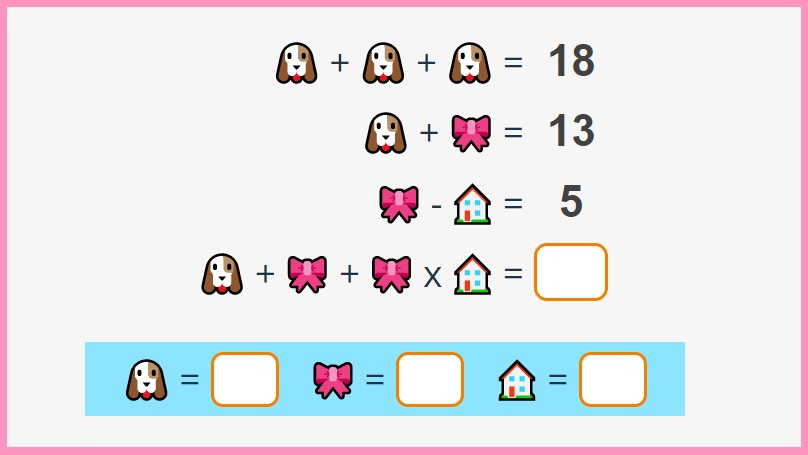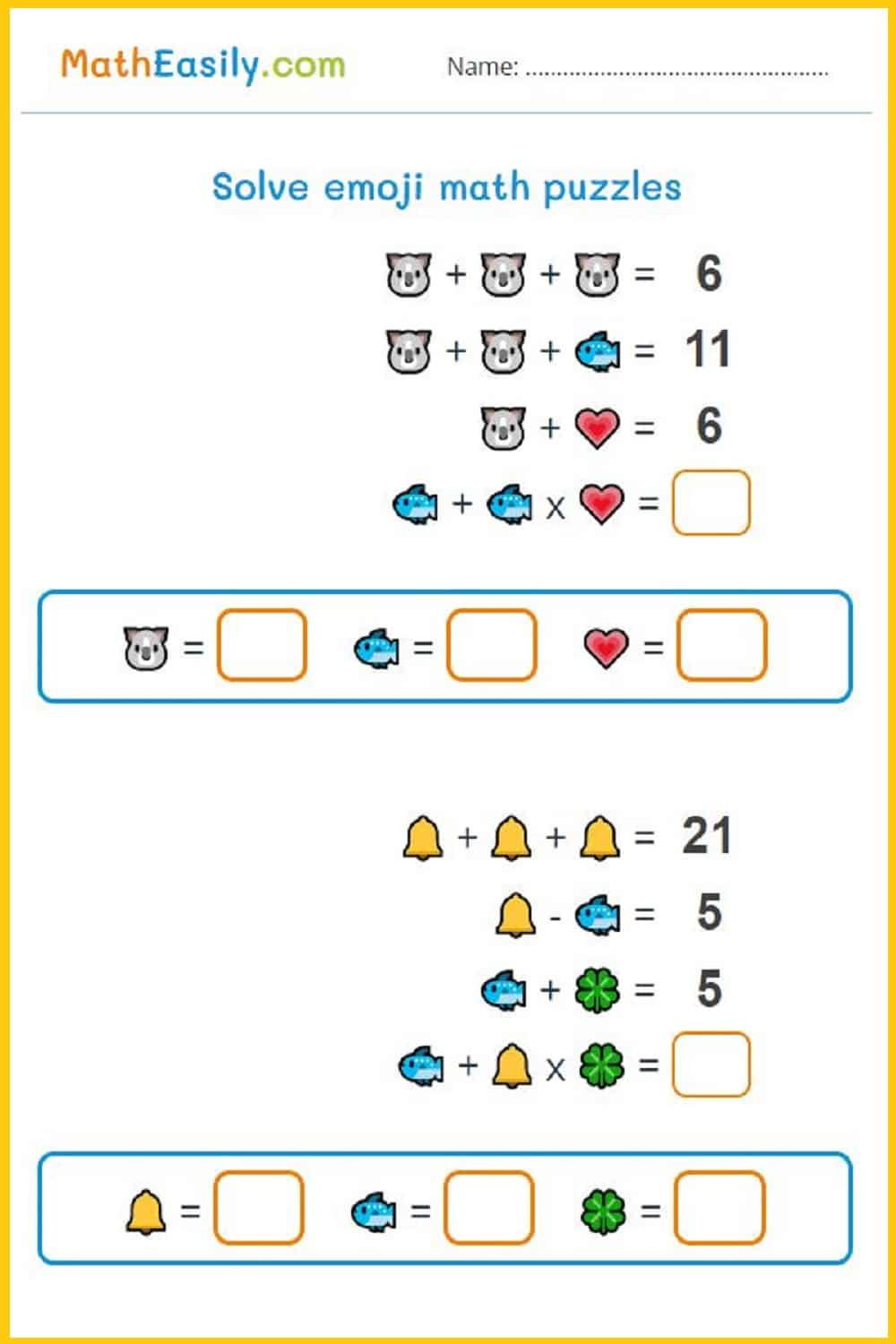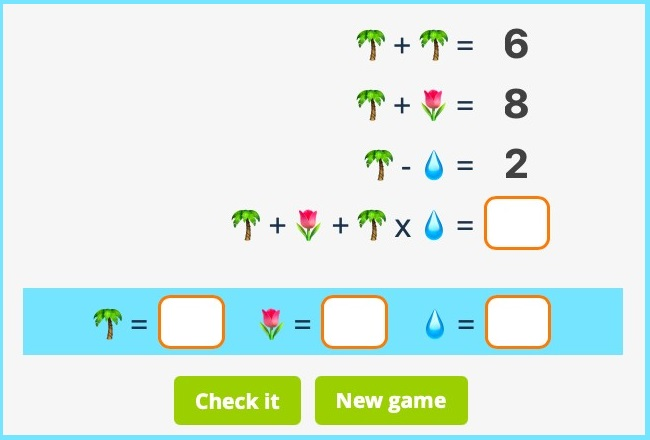# Maths picture puzzles

New gameSolve math picture puzzles online.
Click ´Check it´ to see answers.Sometimes is for kids more convenient to solve emoji math puzzles on printable worksheet. They can make notes on the paper and solve these algebra puzzles more easily.

So, I prepared printable math picture puzzles, which you can generate according the choosen level: just click the link ´Generate a new PDF´. This way every student in the classroom will have their own emoji puzzle in PDF:

### Math picture puzzle### Picture math puzzles with answers PDF

Or you can download prepared printable maths picture puzzles with answers in PDF below. Math equation puzzle worksheets are prepared in 3 levels: easy for Grades 1-2, medium difficulty level for Grades 2-3 and tricky for Grade 3-4. These picture maths puzzles with answers contain also the answer key on the second page.

## Math picture puzzles with answers

Learn addition, subtraction and multiplication with our online maths picture puzzles. You can choose the level of math equation puzzles you want to solve. Click the button ´Check it´ to see emoji logic puzzles answer key.

• ### Easy math picture puzzles

Level 1: The easiest maths picture puzzles help kids to learn basic equations. Because these math picture equation puzzles don´t contain multiplication they are appointed for 1st graders and 2nd graders.

• ### Picture equation puzzles incl. multiplication

Level 2: These emoji maths puzzles are prepared for kids who already started with multiplication. When the equation does not contain parentheses, multiplication takes precedence over addition and subtraction.

• ### Tricky maths picture puzzles with answers

Level 3: Picture logic puzzles for 3rd graders and 4th graders. These algebra picture puzzles contain multiplication and division problems and larger numbers.

## Solve emoji puzzles (with answers included)

You can solve emoji puzzles online or you can download maths emoji puzzles with answers in PDF.

These online emoji puzzles are designed in a way that allows children to work at their own level. The child can start from level 1 and move to levels 2 and 3 for more difficult math picture equations puzzles and can get rid of the fear. Their problem-solving skills will improve, and they begin to understand the structure of maths emoji puzzles and apply their thinking to the new maths picture puzzle.

### How to solve emoji puzzles online?

Look at the picture of the emoji logic puzzle below:

Row No. 1: if the total of two trees are 6, one tree is equal to 3 (3+3=6).

Row No. 2: there is a tree and a flower. We already know that the value of one tree is 3. How much more do we need to add on 3 to make it 8? Right FIVE. Now we know that the value of the flower is equal to 5.

Row No. 3: there is one tree and one water drop. The value of the tree is 3, and we want '2' as an answer. Which number should we subtract from '3' so that we can get 2? It is 1, because: Tree - Water drop (3 - 1 = 2). The value of the water drop is 1.Let's complete the last step. First, you need to know one rule of maths. When there is more than one mathematical symbol in a question, we always do multiplication/division before addition/subtraction.

Row No. 4: for the last row, we will first multiply the tree and the water-drop. Why? Because we always do multiplication before addition. Tree (3) x Water drop (1) = 3. Let's add to this result the value flower and tree. The value of the flower is 5, and the value of the tree is 3. 3+5+3 = 11. The final answer is 11.

You can also learn how to solve math equation puzzles in this tutorial on YouTube.

## Math picture equations puzzles

Picture math puzzles are created in 3 levels, so children can start to solve easy picture logic puzzles.

When kids understand the solution procedure, they can continue to solve more tricky maths picture puzzles with multiplication and division tasks.

If you like these picture equation puzzles you will enjoy Christmas math puzzles during festive Holiday time.## Math equation puzzles

Math picture equation puzzles with answers help kids to learn math equations in a fun way. Solving equations puzzles is fun and exciting.

If you like math picture puzzles try also these online math puzzles: addition puzzles, subtraction puzzles, multiplication puzzles and division puzzles.

## Emoji maths puzzles with answers

Emoji math puzzles are for kids easy to understand. You can solve picture maths puzzles online on any device or you can download our printable emoji logic puzzles in PDF.

If kids have managed to solve this maths emoji puzzles, then they can easily learn to solve more complex mathematical puzzles.